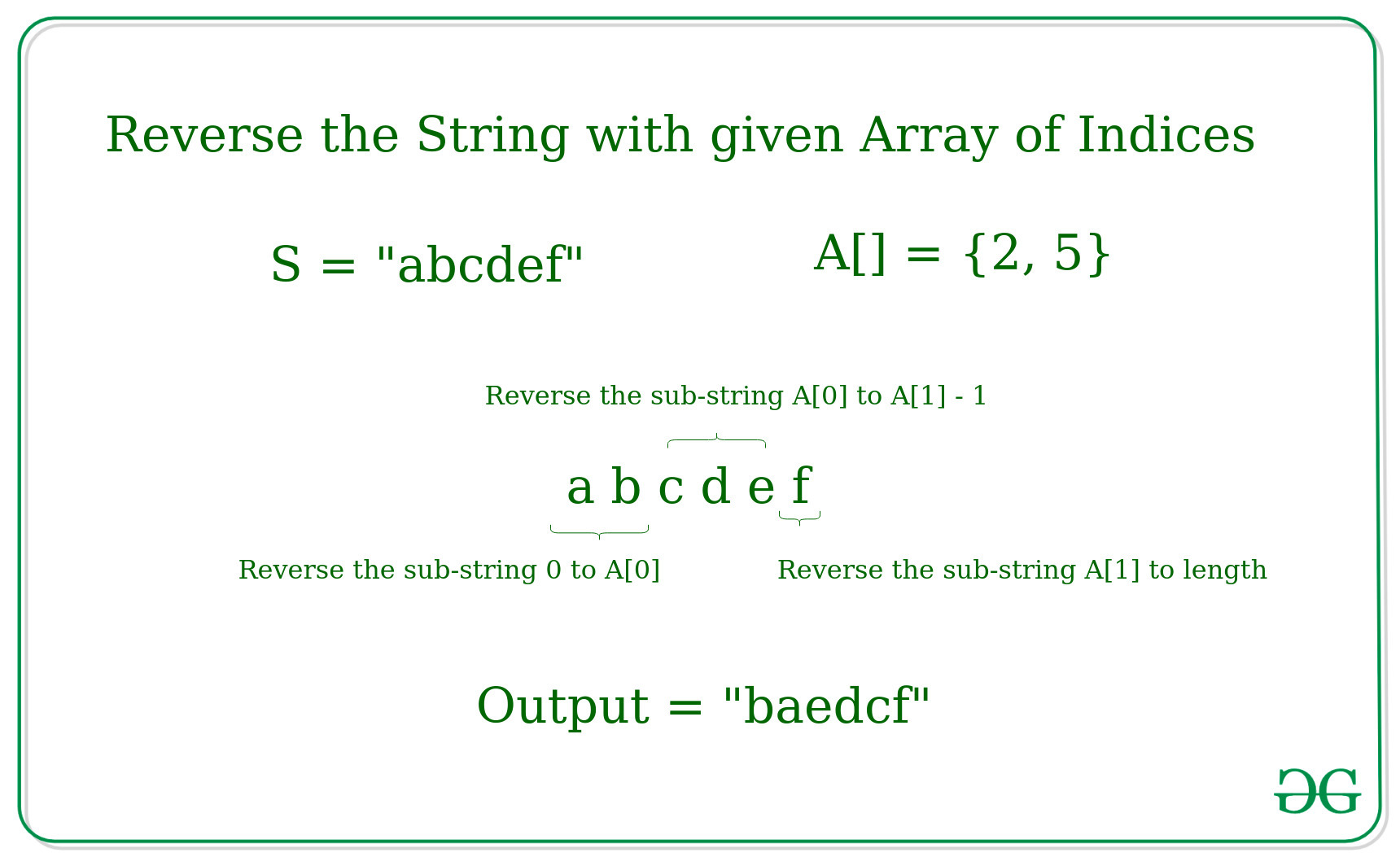Open in App
Not now

# Reverse the substrings of the given String according to the given Array of indices

• Difficulty Level : Basic
• Last Updated : 06 Jan, 2023

Given a string S and an array of indices A[], the task is to reverse the substrings of the given string according to the given Array of indices.
Note: A[i] ≤ length(S), for all i.
Examples:

Input: S = “abcdef”, A[] = {2, 5}
Output: baedcf
Explanation:Input: S = “abcdefghij”, A[] = {2, 5}
Output: baedcjihgf

Approach: The idea is to use the concept of reversing the substrings of the given string.

• Sort the Array of Indices.
• Extract the substring formed for each index in the given array as follows:
• For the first index in the array A, the substring formed will be from index 0 to A (exclusive) of the given string, i.e. [0, A)
• For all other index in the array A (except for last), the substring formed will be from index A[i] to A[i+1] (exclusive) of the given string, i.e. [A[i], A[i+1])
• For the last index in the array A, the substring formed will be from index A[i] to L (inclusive) where L is the length of the string, i.e. [A[i], L]
• Reverse each substring found in the given string

Below is the implementation of the above approach.

## C++

 `// C++ implementation to reverse``// the substrings of the given String``// according to the given Array of indices` `#include ``using` `namespace` `std;` `// Function to reverse a string``void` `reverseStr(string& str,``                ``int` `l, ``int` `h)``{``    ``int` `n = h - l;` `    ``// Swap character starting``    ``// from two corners``    ``for` `(``int` `i = 0; i < n / 2; i++) {``        ``swap(str[i + l], str[n - i - 1 + l]);``    ``}``}` `// Function to reverse the string``// with the given array of indices``void` `reverseString(string& s, ``int` `A[], ``int` `n)``{` `    ``// Reverse the string from 0 to A``    ``reverseStr(s, 0, A);` `    ``// Reverse the string for A[i] to A[i+1]``    ``for` `(``int` `i = 1; i < n; i++)``        ``reverseStr(s, A[i - 1], A[i]);` `    ``// Reverse String for A[n-1] to length``    ``reverseStr(s, A[n - 1], s.length());``}` `// Driver Code``int` `main()``{``    ``string s = ``"abcdefgh"``;``    ``int` `A[] = { 2, 4, 6 };``    ``int` `n = ``sizeof``(A) / ``sizeof``(A);` `    ``reverseString(s, A, n);``    ``cout << s;` `    ``return` `0;``}`

## Java

 `// Java implementation to reverse``// the subStrings of the given String``// according to the given Array of indices``class` `GFG``{` `static` `String s;` `// Function to reverse a String``static` `void` `reverseStr(``int` `l, ``int` `h)``{``    ``int` `n = h - l;` `    ``// Swap character starting``    ``// from two corners``    ``for` `(``int` `i = ``0``; i < n / ``2``; i++)``    ``{``        ``s = swap(i + l, n - i - ``1` `+ l);``    ``}``}` `// Function to reverse the String``// with the given array of indices``static` `void` `reverseString(``int` `A[], ``int` `n)``{` `    ``// Reverse the String from 0 to A``    ``reverseStr(``0``, A[``0``]);` `    ``// Reverse the String for A[i] to A[i+1]``    ``for` `(``int` `i = ``1``; i < n; i++)``        ``reverseStr(A[i - ``1``], A[i]);` `    ``// Reverse String for A[n-1] to length``    ``reverseStr(A[n - ``1``], s.length());``}``static` `String swap(``int` `i, ``int` `j)``{``    ``char` `ch[] = s.toCharArray();``    ``char` `temp = ch[i];``    ``ch[i] = ch[j];``    ``ch[j] = temp;``    ``return` `String.valueOf(ch);``}` `// Driver Code``public` `static` `void` `main(String[] args)``{``    ``s = ``"abcdefgh"``;``    ``int` `A[] = { ``2``, ``4``, ``6` `};``    ``int` `n = A.length;` `    ``reverseString(A, n);``    ``System.out.print(s);``}``}` `// This code is contributed by Rajput-Ji`

## Python3

 `# Python3 implementation to reverse``# the substrings of the given String``# according to the given Array of indices` `# Function to reverse a string``def` `reverseStr(``str``, l, h):``    ``n ``=` `h ``-` `l` `    ``# Swap character starting``    ``# from two corners``    ``for` `i ``in` `range``(n``/``/``2``):``        ``str``[i ``+` `l], ``str``[n ``-` `i ``-` `1` `+` `l] ``=` `str``[n ``-` `i ``-` `1` `+` `l], ``str``[i ``+` `l]` `# Function to reverse the string``# with the given array of indices``def` `reverseString(s, A, n):` `    ``# Reverse the from 0 to A``    ``reverseStr(s, ``0``, A[``0``])` `    ``# Reverse the for A[i] to A[i+1]``    ``for` `i ``in` `range``(``1``, n):``        ``reverseStr(s, A[i ``-` `1``], A[i])` `    ``# Reverse String for A[n-1] to length``    ``reverseStr(s, A[n ``-` `1``], ``len``(s))` `# Driver Code``s ``=` `"abcdefgh"``s ``=` `[i ``for` `i ``in` `s]``A ``=` `[``2``, ``4``, ``6``]``n ``=` `len``(A)` `reverseString(s, A, n)``print``("".join(s))` `# This code is contributed by mohit kumar 29`

## C#

 `// C# implementation to reverse``// the subStrings of the given String``// according to the given Array of indices``using` `System;` `class` `GFG``{` `static` `String s;` `// Function to reverse a String``static` `void` `reverseStr(``int` `l, ``int` `h)``{``    ``int` `n = h - l;` `    ``// Swap character starting``    ``// from two corners``    ``for` `(``int` `i = 0; i < n / 2; i++)``    ``{``        ``s = swap(i + l, n - i - 1 + l);``    ``}``}` `// Function to reverse the String``// with the given array of indices``static` `void` `reverseString(``int` `[]A, ``int` `n)``{` `    ``// Reverse the String from 0 to A``    ``reverseStr(0, A);` `    ``// Reverse the String for A[i] to A[i+1]``    ``for` `(``int` `i = 1; i < n; i++)``        ``reverseStr(A[i - 1], A[i]);` `    ``// Reverse String for A[n-1] to length``    ``reverseStr(A[n - 1], s.Length);``}` `static` `String swap(``int` `i, ``int` `j)``{``    ``char` `[]ch = s.ToCharArray();``    ``char` `temp = ch[i];``    ``ch[i] = ch[j];``    ``ch[j] = temp;``    ``return` `String.Join(``""``,ch);``}` `// Driver Code``public` `static` `void` `Main(String[] args)``{``    ``s = ``"abcdefgh"``;``    ``int` `[]A = { 2, 4, 6 };``    ``int` `n = A.Length;` `    ``reverseString(A, n);``    ``Console.Write(s);``}``}` `// This code is contributed by 29AjayKumar`

## Javascript

 ``

Output:

`badcfehg`

Time Complexity: O(l * n), where l is the length of the given string and n is the size of the given array.
Auxiliary Space: O(1), no extra space is required, so it is a constant.

My Personal Notes arrow_drop_up